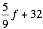1. Which of the following Python identifiers are legal variable names? For those that are not, say why not.
1. x
2. Celsius
3. spam3
4. <hello>
5. question?
Answer: Choices a, b, and c are legal variable names. Choice d is not because the characters “<” and “>” are not allowed in variable names; neither is “?”, so choice e is not.
2. What is the data type (int, long int, float, string, list) of the following:
1. 3
2. 3.0
3. 3L
4. "hello"
5. 'x'
6. [ 1, 2, 3 ]
1. int
2. float
3. long int
4. string
5. string
6. list
3. Write the following formulas in Python:
1.2. 7 × 9
3. 42
1. (5.0 / 9.0) * f + 32
2. 7 * 9
3. 4 ** 2
4. What does the following program print?
for i in "hello":
print i, "-->", chr(ord(o)+1);
h --> i
e --> f
l --> m
l --> m
o --> p
5. What does the following program print?
for i in [1, 7, 4]:
x = (-1) ** i * i;
print i, x;
1 -1
7 -7
4 4
6. When I ran the following program:
x = input("Enter a number: ");
y = 1.0 / x;
print "The reciprocal of", x, "is", y;
I got the following error message:
Traceback (most recent call last):
File "test.py", line 3, in <module>
y = 1 / x;
ZeroDivisionError: integer division or modulo by zero
What caused the error? Please be specific.
Answer: The problem is that there was an attempt to divide by 0. This occurred on line 2. As there is a division by x there, and I entered the value of x in response to the prompt (see line 1), I obviously entered 0.